Next: TIME-FREQUENCY-STATISTICAL RESOLUTION Up: Resolution Previous: TIME-STATISTICAL RESOLUTION

# FREQUENCY-STATISTICAL RESOLUTION

Observations of sea level for a long period of time can be summarized in terms of a few statistical averages such as the mean height m and the variance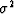.Another important kind of statistical average for use on such geophysical time series is the power spectrum. Some mathematical models explain only statistical averages of data and not the data themselves. In order to recognize certain pitfalls and understand certain fundamental limitations on work with power spectra, we first consider an idealized example.

Let xt be a time series made up of independently chosen random numbers. Suppose we have n of these numbers. We can then define the data sample polynomial X(Z)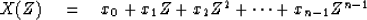(38)
We can now make up a power spectral estimate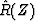from this sample of random numbers by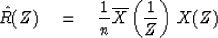(39)
The difference between this and our earlier definition of spectrum is that a power spectrum has the divisor n to keep the expected result from increasing linearly with the somewhat arbitrary sample size n.

The definition of power spectrum is the expected value of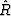, namely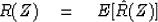(40)
It might seem that a practical definition would be to let n tend to infinity in (39). Such a definition would lead us into a pitfall which is the main topic of the present section. Specifically, from Figure 3 we conclude thatis a much fuzzier function than R(Z), so that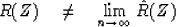(41)

 4-2 Figure 3 Amplitude spectra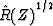of samples of n random numbers. These functions seem to oscillate over about the same range for n = 512 as they do for n = 32. As n tends to infinity we expect infinitely rapid oscillation.To understand why the spectrum is rough, we identify coefficients of like powers of Z in (39). We have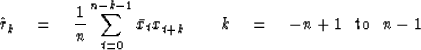(42)

enabling us to write (39) for real time series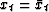as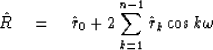(43)
Let us examine (43) for large n. To do this, we will need to know some of the statistical properties of the random numbers. Let them have zero mean m = E(xt) = 0 and let them have known constant variance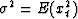and recall our assumption of independence which means that E(xt xt+ s) = 0 if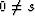.Because of random fluctuations, we have learned to expect that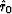will come out to beplus a random fluctuation component which decreases with sample size as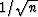,namely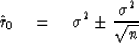(44)
Likewise,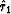should come out to be zero but the definition (42) leads us to expect a fluctuation component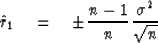(45)
For the kth correlation value k > 1 we expect a fluctuation of order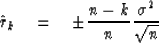(46)
An autocorrelation of a particular set of random numbers is displayed in Figure 4.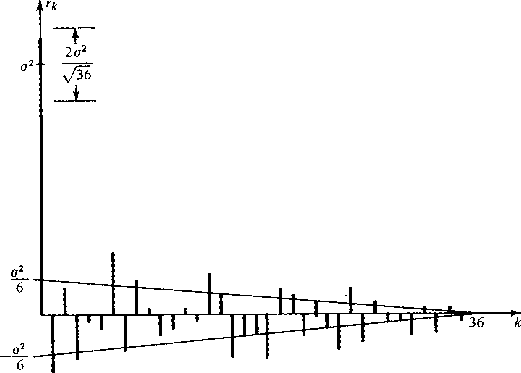4-3
Figure 4
Positive lags of autocorrelation of 36 random numbers.

Now one might imagine that as n goes to infinity the fluctuation terms vanish and (39) takes the limiting form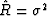.Such a conclusion is false. The reason is that although the individual fluctuation terms go asthe summation in (43) contains n such terms. Luckily, these terms are randomly canceling one another so the sum does not diverge as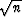.We recall that the sum of n random signed numbers of unit magnitude is expected to add up to a random number in the range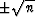.Thus the sum (43) adds up to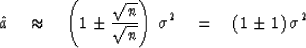(47)
This is the basic result that a power spectrum estimated from the energy density of a sample of random numbers has a fluctuation from frequency to frequency and from sample to sample which is as large as the expected spectrum.

It should be clear that letting n go to infinity does not take us to the theoretical result.The problem is that, as we increase n, we increase the frequency resolution but not the statistical resolution. To increase the statistical resolution we need to simulate ensemble averaging. There are two ways to do this:  (1) Take the sample of n points and break it into k equal-length segments of n/k points each. Compute an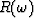for each segment and then add all k of thetogether, or (2) formfrom the n-point sample. Of the n/2 independent amplitudes, replace each one by an average over its k nearest neighbors. Whichever method, (1) or (2), is used it will be found that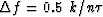and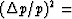inverse of number of degrees of freedom averaged over = 1/k. Thus, we have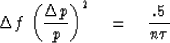If some of the data are not used, or are not used effectively, we get the usual inequality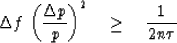Thus we see that, if there are enough data available (n large enough), we can get as good resolution as we like. Otherwise, improved statistical resolution is at the cost of frequency resolution and vice versa.

We are right on the verge of recognizing a resolution tradeoff, not only between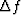and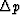but also with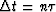,the time duration of the data sample. Recognizing now that the time duration of our data sample is given by,we obtain the inequality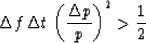(48)
The inequality will be further interpreted and rederived from a somewhat different point of view in the next section.

In time-series analysis we have the concept of coherency which is analogous to the concept of correlation defined in Sec. 4-2. There we had for two random variables x and y that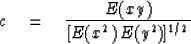Now if xt and yt are time series, they may have a relationship between them which depends on time-delay, scaling, or even filtering. For example, perhaps Y(Z) = F(Z) X(Z) + N(Z) where F(Z) is a filter and nt is unrelated noise. The generalization of the correlation concept is to define coherency by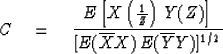Correlation is a real scalar. Coherency is complex and expresses the frequency dependence of correlation. In forming an estimate of coherency it is always essential to simulate some ensemble averaging. Note that if the ensemble averaging were to be omitted, the coherency (squared) calculation would give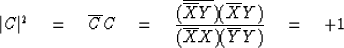which states that the coherency squared is +1 independent of the data. Because correlation scatters away from zero we find that coherency squared is biased away from zero.

 4-4 Figure 5 Model of random time series generation.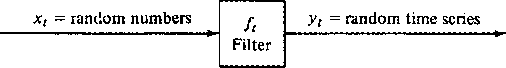Next: TIME-FREQUENCY-STATISTICAL RESOLUTION Up: Resolution Previous: TIME-STATISTICAL RESOLUTION
Stanford Exploration Project
10/30/1997